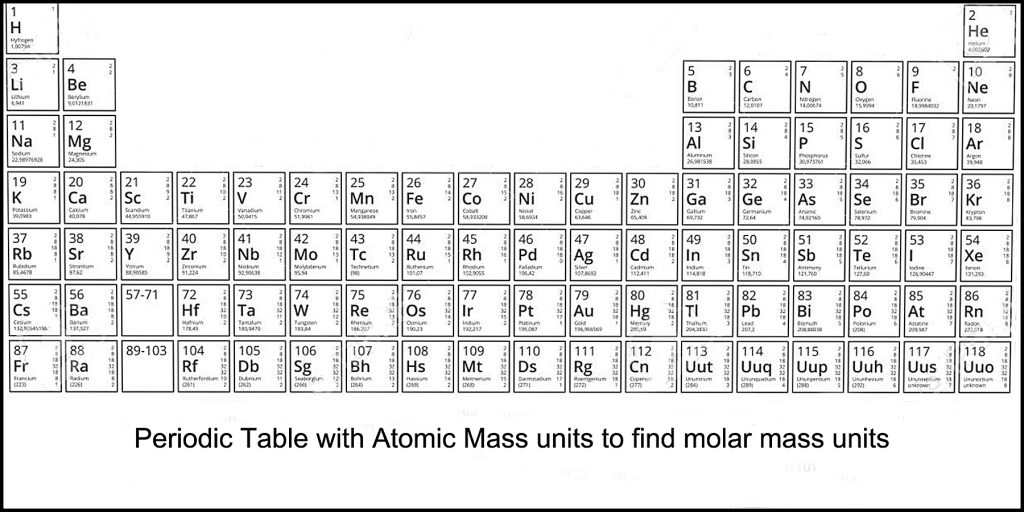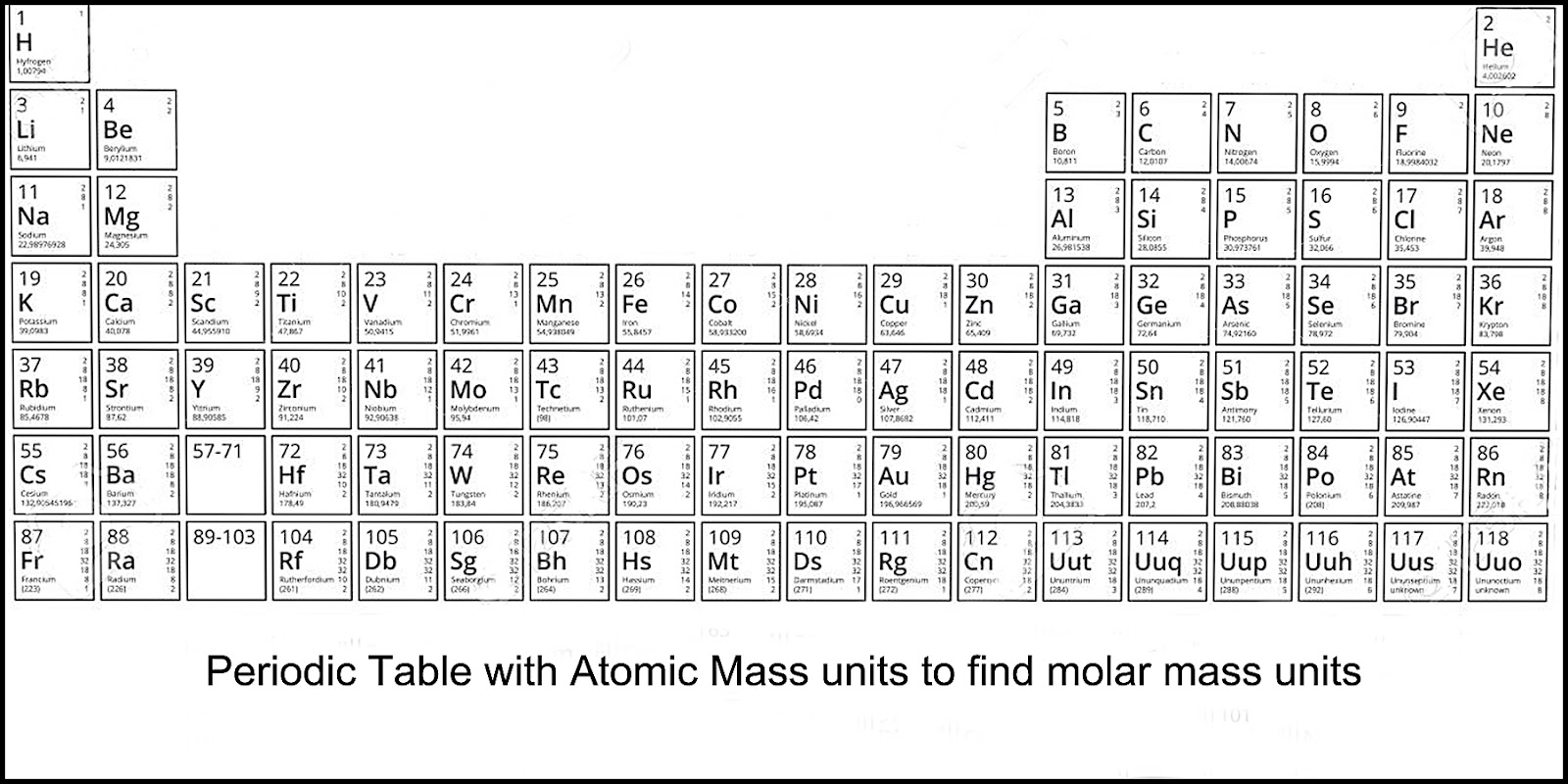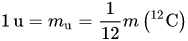# Mass, Weight, Gravity and Atomic Number

The mass of an object is a measure of the object's amount of matter. The weight of an object is a measure of the force exerted on the object by gravity. The pull of gravity on the earth gives an object a downward acceleration of about 9.8 m/second squared. Atomic mass also known as atomic weight is the weighted average mass of an atom of an element based on the relative abundance of that element's isotopes. The Atomic Number is a count of the total number of protons in an atom's nucleus. This is found at the top of each atom and is usually the same as the number of electrons.The Atomic Mass number is the total mass of protons,  neutrons and electrons using molar mass. You can find that number in the bottom portion in each element in the periodic table. That number represents the number of amu or number of daltons.That’s a lot of stuff to understand so let’s dig in and simplify this subject. I’m not a mathematician so this info is for the layman or interested newbie.

The unit of mass (amu) used to express atomic and molecular weights is equal to one-twelfth of the mass of an atom of carbon-12. It is equal to approximately 1.66 x 10^-24 gms. The dalton (Da) is the new name for the unified atomic mass unit (amu). The symbol for amu was = u.

Now, in 2019 the new symbol is da (dalton). For instance, 2 x 1.66 x 10^24 grams is 2 daltons or 2 x amu.

On Earth, a 1 kg object weighs 9.8 N (Newtons), so to find the weight of an object in N simply multiply the mass by 9.8 N. Or, to find the mass in kg, divide the weight in Newtons  by 9.8 N.

## Examples using a Proton

A proton’s mass is the same as a hydrogen atom without the electron and it is 1.00727647 atomic mass units (amu). Therefore, it’s mass is equal to approximately, 1.00727647 (amu) x 1.66 x 10^-24 grams per amu. So a proton weighs about 1.66 x 10^-24 grams.

Protons have an internal structure of three particles (quarks) held together by attractive forces (gluons). Naive assumptions would give each quark 1/3 proton mass.

But, about 95 percent to 98 percent of a proton mass is not from the quark mass. Most of the proton mass is derived from the energy between the quarks. Remember, “E = mc^2” the energy equivalent is measured as mass.

Because each proton and each neutron weighs about one atomic mass unit (amu). By adding together the number of protons and neutrons and multiplying by 1 amu, you can calculate the approximate mass of an atom.

For example, the carbon 12 atom has a mass of 12 da (daltons) or as 12 amu which is 12 x 1.66053 x 10^-24 grams = 1.992646547 x 10^-23 grams.

## Examples using Hydrogen

To make things simpler a molar mass is the weight of 1 mole (6.022 x 10^23 units) of a substance which equals the Atomic Mass from the periodic table. For hydrogen that equals 1.008 grams.

Note that hydrogen atoms have an electron to balance proton charge. Protons without electrons, either as dissolved in solution or as plasma, are very different from hydrogen atoms.

Since the calculation does not stop there, we can ignore the physical absurdity in pretending electrons don’t exist.

Keep in mind that a “molar mass” calculation method can be done with any element. Look up the Atomic Mass of an atom in the periodic table. This number is the molar mass in grams of one mole.

For example, Nitrogen has an atomic mass of 14 grams for one mole of nitrogen.

Gold has an atomic mass of 196.967 amu, so one mole of gold has a mass of 196.967 grams.

However, sources of error creep up when using hydrogen. Each hydrogen atom contains only one proton, so one mole of protons weighs 1.0079 g (the atomic mass from periodic table).

First, protons in hydrogen atoms are not bonded. In other elements, protons are bonded to neutrons. Protons bonded in a nucleus have less energy—therefore slightly less mass—than isolated protons.

Second, electron number, and the error if ignoring them, begins to add up. Accounting for electrons makes the whole endeavor more complicated.

The number of units in one mole of any substance is equal to 6.022140857 × 10^23 particles. The units may be electrons, atoms, ions, or molecules, depending on the nature of the substance and the character of the reaction.

The specific number of molecules in one gram- mole of a substance, is 6.022140857 × 10^23, a quantity called Avogadro's Number or the Avogadro constant.

For example, the molecular weight of oxygen is 32.00, so that one gram-mole of oxygen has a mass of 32.00 grams and contains 6.022140857 × 10^23 molecules.

Or, one gram- mole of oxygen atoms is 16.00 grams and also has the same number of units 6.022 x 10^23 (Avogadro number).

Avogadro’s law, a statement that under the same conditions of temperature and pressure, equal volumes of different gases contain an equal number of molecules. The law is approximately valid for real gases at sufficiently low pressures and high temperatures.

The volume occupied by one gram-mole of gas is about 22.4 litres (0.791 cubic feet) at standard temperature and pressure (0 °C, 1 atmosphere) and is the same for all gases, according to Avogadro’s law.

## Key Takeaways

• The mole allows scientists to calculate the number of elementary entities (usually atoms or molecules ) in a certain mass of a given substance.

• Avogadro’s number is an absolute number: there are 6.022×1023 elementary entities in 1 mole.

• The mass of one mole (molar mass) of a substance is equal to that substance’s molecular weight in grams.

•  For example, the mean molecular weight of water is 18.015 atomic mass units (amu), so one mole of water has a weight of 18.015 grams.

If you resonate with this new information I want you to be on my tribe of followers. I would like to encourage your dreams and confirm your thoughts that science will show us the Ultimate Truth. I care about your evolution as you start to wake up to a new paradigm.

Your influence is greater than you can imagine! Join my list of subscribers and get my latest post in your email. Thanks for being here. As always, be well. https://lovinthings.com

### About the Author Erik Lovin

Erik has a BSc degree and is a retired professional photographer who is now a published author of many books. His passion is understanding how life and the universe work. He is currently blogging about the science of the Big Bang and science in your life. Erik is helping his tribe with questions about the universe. His goal is to help find a theory of everything (TOE). In order to do that, he is trying to prove light has mass and that the fabric of spacetime is a false theory. We are welcoming questions and answers that you might have about the universe.September 14, 2019

I think this is among the most vital info for me. And i am glad reading your article. But should remark on few general things, The site style is ideal, the articles is really excellent : D. Good job, cheersErik Lovin says September 14, 2019

Hi thank you for your kind words. I happy that you enjoyed it. cheersDeniseBig says August 9, 2019

Hi. I have checked your lovinthings.com and i see you’ve got some duplicate
content so probably it is the reason that you don’t rank
hi in google. But you can fix this issue fast.
There is a tool that generates content like human, just search in google: miftolo’s toolsErik Lovin says August 9, 2019

Thanks for the comment and for your tip. Cheers.